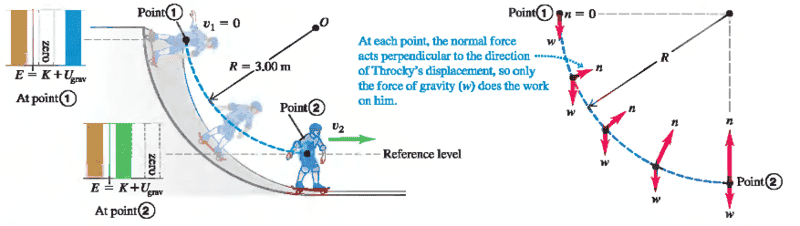# Circular motion and Net force

ShizukaSm

## Homework StatementIn my physics book (Young and Freedman 12 edition), in the chapter about conservation of energy, it teaches me that I can use the theory of conservation of energy and discover the velocity at the bottom of a circular path, having the height of this circular path. I understand and am ok with that part, however, there's another thing that I don't understand.

Suppose this image, if we calculate the velocity at the bottom, and then the net force, we will discover that the normal force is in fact applying 735 Newtons. Well, here's the thing, as soon as the circular paths ends and the "straight" path begins, the normal force will be simply equal to the weight again(245N), why does this happen? What I mean is, what in the circle magically makes the normal increase this much?